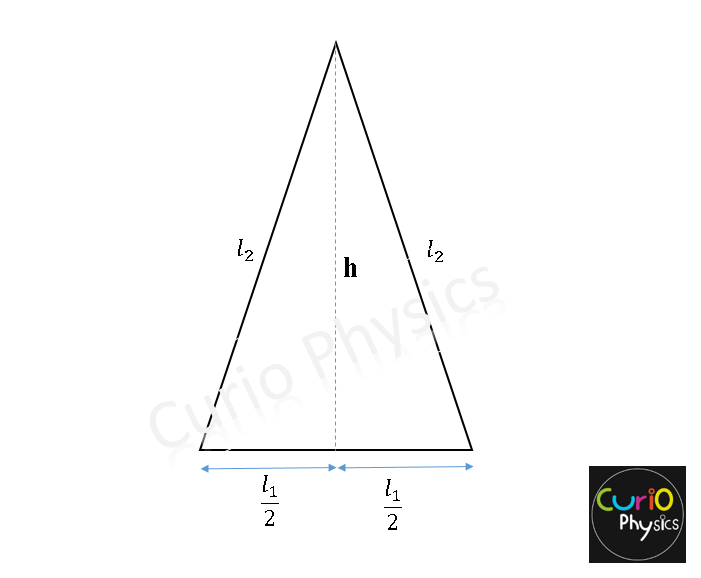# Numerical on Thermal Expansion

##### Numerical on Thermal Expansion

Q1. A metal rod A of length 25 cm expands by 0.05 cm, when its temperature is raised from 0°C to 100°C. Another rod B of a different metal of length 40 cm expands by 0.04 cm for the same rise in temperature. A third rod C of length 50 cm made up of pieces of rods A and B placed end to end expands by 0.03 cm on heating from 0°C to 50°C. Find the length of each portion of the composite Road.

Solution. For Rod A :-

0.05 = 25 αA (100)

⇒    αA = 2×10-5 ºC-1     ……….(1)

Also for rod B :-

0.04 = 40 αB (100)

⇒     αB = 10-5 ºC-1      ……….(2)

Now for 3rd composite rod C :-

lA + lB = 50cm     ………..(3)

⇒ ΔlA + ΔlB = 0.03

lAαA (50) + lBαB (50) = 0.03

lA × 2 × 10-5 × (50) + lB × 10-5 × (50) = 0.03

⇒ 2lA + lB  = 60    ………..(4)

from (3) and (4), we get

lA = 10 cm  &  lB = 40 cm

Q2. If an isotropic solid has coefficients of linear expansion αX , αY & αZ for three mutually perpendicular directions in the solid, what is the coefficient of volume expansion for the solid?

Solution. Consider a cube of that material with edges parallel to x, y & z axis having side l0  at T = 0ºC.

At a temperature T, the new dimensions of the cube will be :-

lx = l0 [1 + αx T]

ly = l0 [1 + αy T]

lz = l0 [1 + αz T]

So volume of solid,

V  =  l0 [1 + αx T]  ×  l0 [1 + αy T]  ×  l0 [1 + αz T]

⇒      V  =  (l0)3 [1 + αx T] [1 + αy T] [1 + αz T]

As V0  =  (l0)3

⇒      V  =  V0  [1 + αx T] [1 + αy T] [1 + αz T]

⇒      V  =  V0  [1 + (αx  + αy  + αz) T]     …..(1)

[neglecting higher powers of αx , αy  and αz ]

now finally if γ is coefficient of volume expansion of the solid then

V  =  V0  [1 + γ T]    …..(2)

comparing equation (1) and (2), we get

γ = αx  + αy  + αz

Q3. An isosceles triangle is formed with a rod of length  and coefficient of linear expansion  for the base and two thin rods each of length  and coefficient of linear expansion  for the two pieces, if the distance between the apex and the midpoint of the base remain unchanged as the temperatures varied, show that$\displaystyle \frac{{{l}_{1}}}{{{l}_{2}}}=2\sqrt{\frac{{{\alpha }_{2}}}{{{\alpha }_{1}}}}$Solution. First Method :-

The height of the triangle,$\displaystyle h=\sqrt{l_{2}^{2}-\frac{l_{1}^{2}}{4}}$

As the temperature increases, the height remains the same as the old length.$\displaystyle \Rightarrow \sqrt{l_{2}^{2}{{(1+{{\alpha }_{2}}t)}^{2}}-\frac{l_{1}^{2}}{4}{{(1+{{\alpha }_{1}}t)}^{2}}}=\sqrt{l_{2}^{2}-\frac{l_{1}^{2}}{4}}$$\displaystyle \Rightarrow \frac{{{l}_{1}}}{{{l}_{2}}}=2\sqrt{\frac{{{\alpha }_{2}}}{{{\alpha }_{1}}}}$

Second Method :-

As with change in temperature T , h remains constant, hence$\displaystyle \frac{dh}{dT}=0$$\displaystyle \Rightarrow \frac{d({{h}^{2}})}{dT}=0$$\displaystyle \Rightarrow \frac{d}{dT}(l_{2}^{2}-\frac{l_{1}^{2}}{4})=0$$\displaystyle 2{{l}_{2}}\frac{d{{l}_{2}}}{dT}-\frac{2}{4}{{l}_{1}}\frac{d{{l}_{1}}}{dT}=0$$\displaystyle 2{{l}_{2}}\frac{d{{l}_{2}}}{dT}=\frac{2}{4}{{l}_{1}}\frac{d{{l}_{1}}}{dT}$$\displaystyle \Rightarrow {{l}_{2}}\frac{{{l}_{2}}{{\alpha }_{2}}dT}{dT}=\frac{{{l}_{1}}}{4}\frac{{{l}_{1}}{{\alpha }_{1}}dT}{dT}$$\displaystyle 4{{\alpha }_{2}}l_{2}^{2}={{\alpha }_{1}}l_{1}^{2}$$\displaystyle \Rightarrow \frac{{{l}_{1}}}{{{l}_{2}}}=2\sqrt{\frac{{{\alpha }_{2}}}{{{\alpha }_{1}}}}$

Q4. A circular hole in an aluminum plate is 2.54 cm in diameter at 0ºC. What is the diameter when the temperature of the plate is raised to 100ºC. Given αAl = 2.3×10-5 ºC-1.

Solution. Change in diameter is a linear change, hence

DT = D0 (1 + αAlΔT) = 2.54 (1 + 2.3×10-5×100) = 2.5458 cm

Q5. A glass beaker holds exactly 1 liter at 0ºC.

1. What is its volume at 50ºC?
2. If the beaker is filled with mercury at 0ºC, what volume of mercury overflows when the temperature is 50ºC?

Given αg = 8.3×10-6 ºC-1   &   γHg = 1.82×10-4 ºC-1

Solution.

i.  New volume of beaker at 50ºC

V beaker = V0(1 + 3αg ΔT) = (1)[1 + 3×8.3×10-6×50] = 1.001 liter

ii. New volume of mercury at 50ºC is

V Hg = V0(1 + γHg ΔT) = (1)[1 + 1.82×10-4×50] = 1.009 liter

the volume of the mercury which overflows ΔV = (1.009 – 1.001) liter = 0.008 liter = 8 ml

Q6. A 1 meter steel scale is to be prepared such that the millimeter intervals are to be  accurate within 5×10-4 mm at a certain temperature. Determine maximum permissible temperature variation during the ruling of the mm mark. Given αsteel = 13.22×10-6 ºC-1

Solution. Here

Δl = 5×10-4 mm ,   l = 1mm ,    αsteel = 13.22×10-6 ºC-1

Δl = αsteel × l × ΔT

5×10-4 = 13.22×10-6 ×1×ΔT

∴ ΔT = 500/13.22 = 37.82 ºC